# Mixing Index and Power Required for Mixing Newtonian and Non-Newtonian Fluid

Newtonian and Non-Newtonian Fluid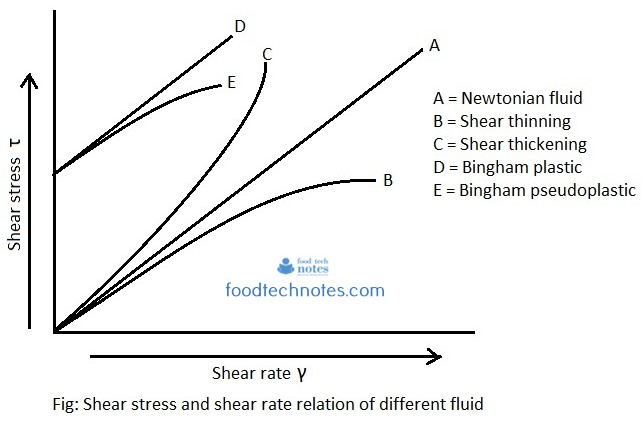Newtonian fluid: For such fluids, viscosity is dependent only on temperature. There is linear increase in shear stress with increasing shear rate. For such fluid, viscosity remain constant no matter how fast they are forced to flow through pipe or channel. Example; water, organic solvent, honey

Bingham plastic: These are the fluid that require minimum stress to be applied before they flow. These are strictly non – Newtonian but once the flow starts, they behave essentially as Newtonian fluid. Example; mayonnaise.

Shear thickening fluid: For such fluid, viscosity increases as shear rate increases. Example; mixture of corn starch and water

Shear thickening fluid: For such fluid, viscosity decreases as shear rate increases. Example; ketchup, paints and blood.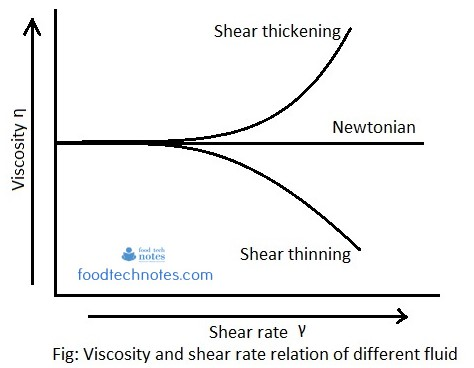Standard deviation in mixing:

During mixing, under ideal condition, sample randomly picked up and analyzed contain same proportion as what is taken initially for mixing. Improper mixing will not have expected composition. Thus the sample analysis is an effective tool to knowing our mixture composition. Calculation of root mean square deviation (σ) from sample will help us to find if complete mixing is achieved. If mixing process is perfect, deviation from mean is minimum, which is nearly zero. Thus standard deviation is zero. In other words, we can say that, smaller the deviation, better the mixing condition. If ‘n’ number of sample is taken of composition X1, X2, X3………Xn, standard deviation for sample is given by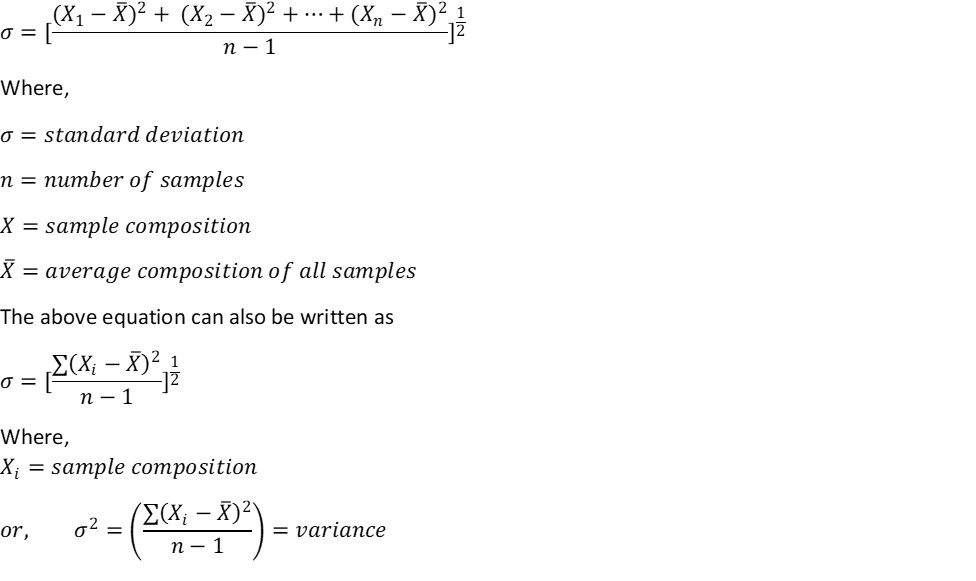Mixing Index :

Solid mixing is conventionally characterized by using principle of probability theory. Let us consider we have two components ‘P’ and ‘Q’ and their relative fractional properties are ‘p’ and ‘q’ respectively.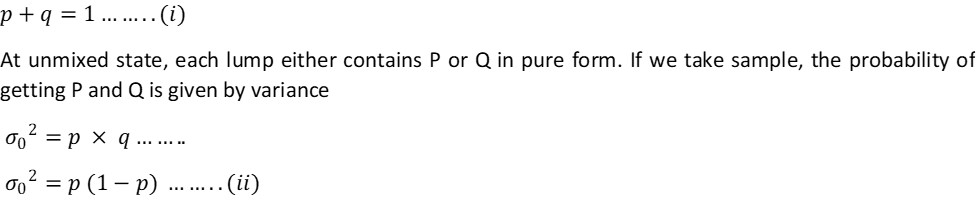If two components are mixed and if we take any one particle, the probability that it is component P is ‘p’ and the probability that it is component Q is ‘q’. Instead of taking one particle, if we take N particle in one lot, probability of occurrence of P and Q is given by variance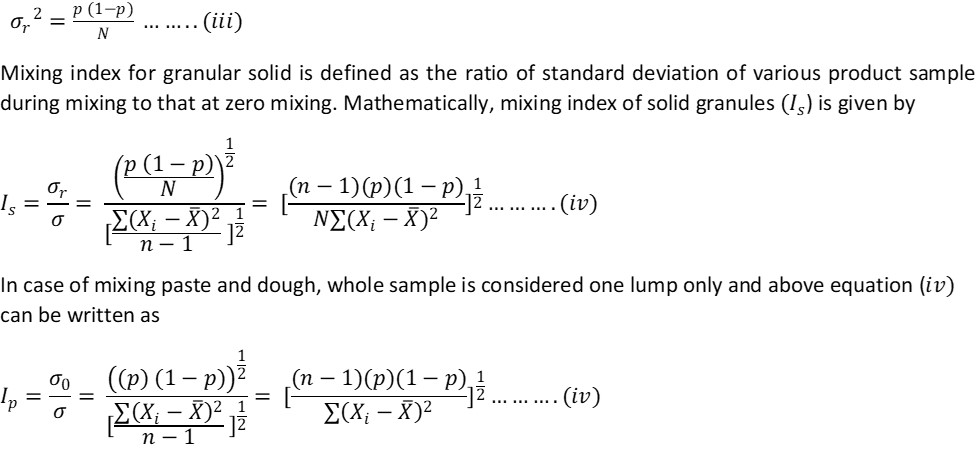Rate of mixing: Rate of mixing at any time under constant condition is proportional to the extent of mixing at that time.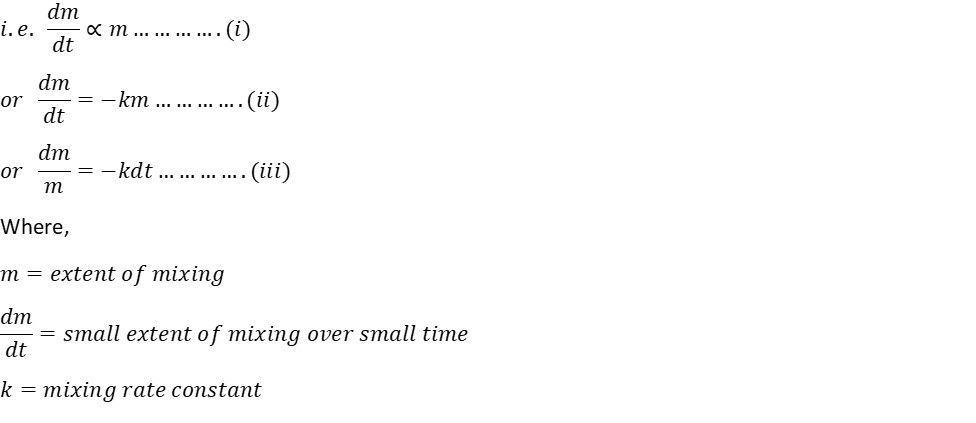Negative sign indicates that rate of mixing falls down as mixing time progress. Integrating above equation within the limit between when t = 0, m = m0 and when t = t and m = m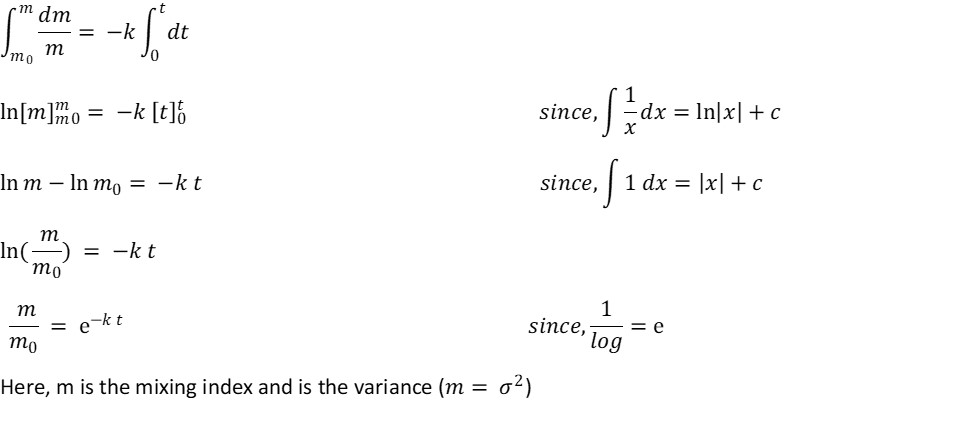Power required for mixing:

Power required for mixing is the evaluation of wattage of power for rotating the impeller when its blade strike and overcome the viscous forces of the liquid broth that give resistance for the rotation of impeller blades. Overcoming of shear resistance gives movement to the impeller blades. Power required by impeller is then the function of

1. Viscosity of fluid
2. Density of fluid
3. Rheological nature of fluid
4. Speed of agitator
5. Type and nature of impeller

However, power calculated doesn’t include electrical or mechanical losses or frictional losses.

Power required for mixing ungassed system: Power required for mixing ungassed system (P0) is a function of fluid properties and agitator speed and is given by relation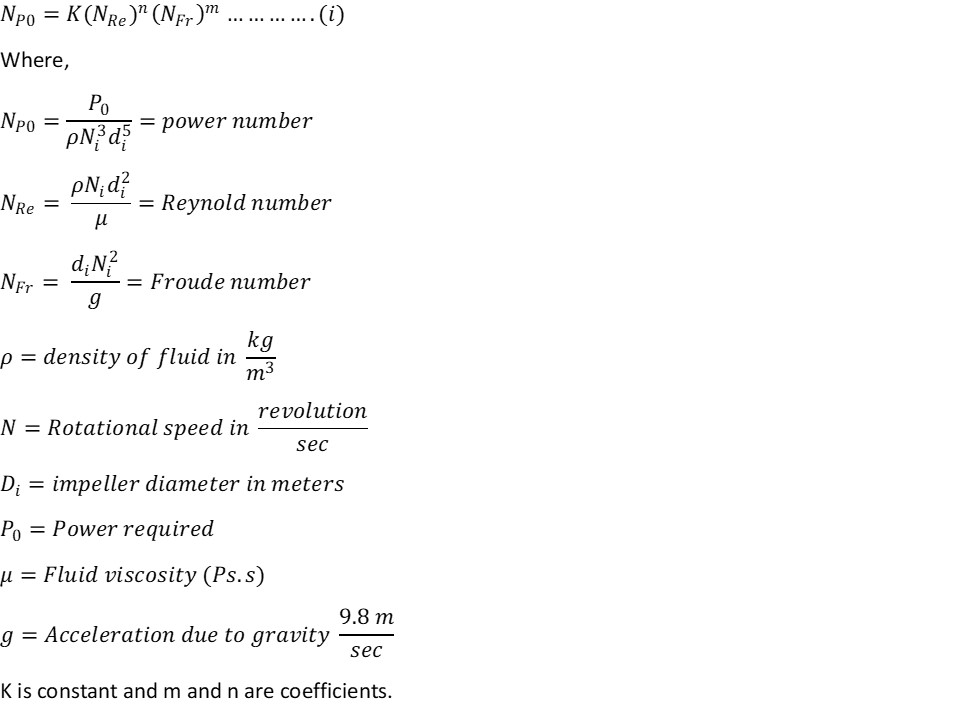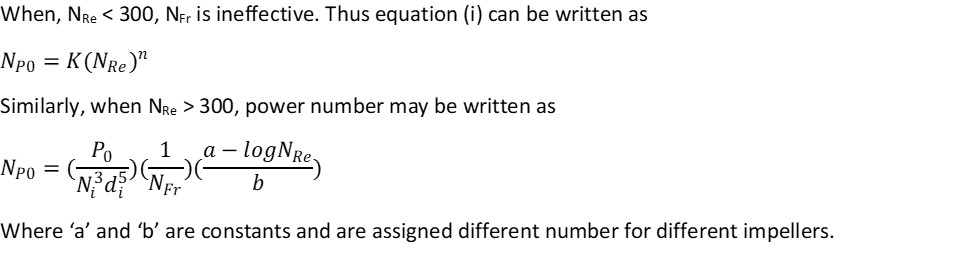Relation between Reynolds number and power number: Mixing of fluid in the mixer may experience different fluid flow during mixing which can be categorized on the basis of their fluid flow.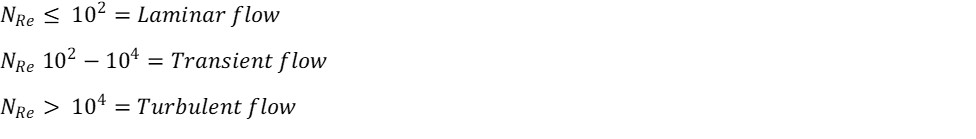If graph is plotted between power number and Reynolds number, the plot can be divided into 3 zones.

Zone I (Laminar Zone): In this zone, power number continuously falls as NRe increases. Here,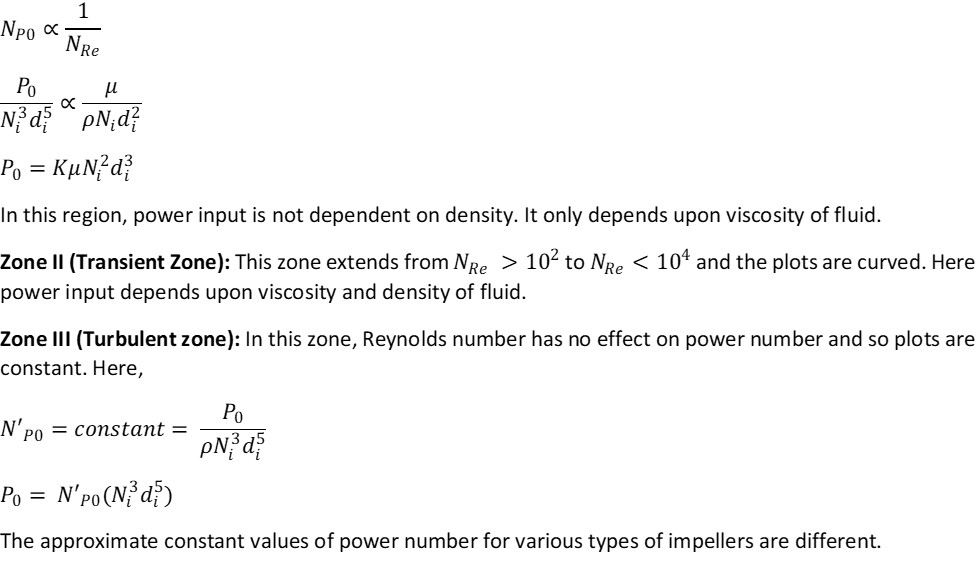The approximate constant values of power number for various types of impellers are different.

Power requirement for non – Newtonian fluid:

Most of the fluid materials and emulsions are non- Newtonian in nature. Non- Newtonian fluids are characterized by the fact that they change their viscosity as the shear rate changes. The term apparent viscosity (μa) is used in place of μ. Apparent viscosity is defined as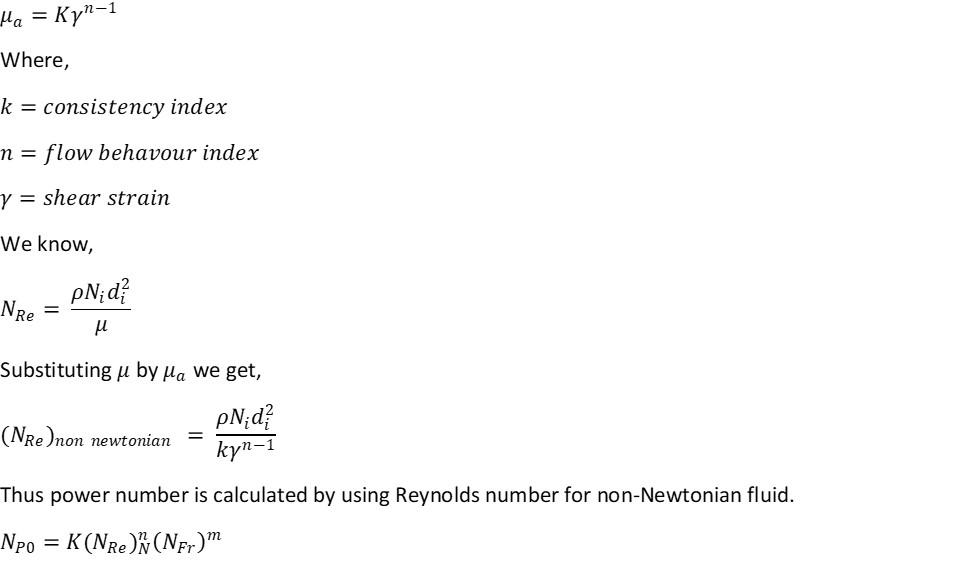Generally it is found that in the laminar zone and turbulent zone power number for Newtonian and non- Newtonian fluids are virtually the same. However in transition zone, power number for non- Newtonian fluid is slightly lower than that for Newtonian fluids.